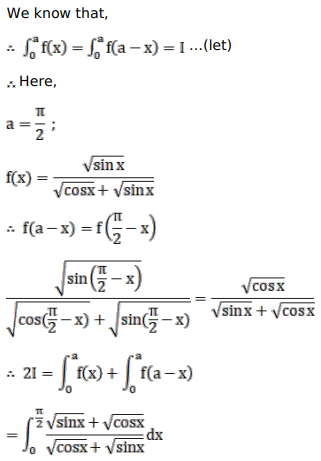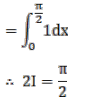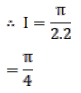# Mark against the correct answer in the following:Question:

Mark $(\sqrt{ })$ against the correct answer in the following:

$\int_{0}^{\pi / 2} \frac{\sqrt{\cos x}}{(\sqrt{\cos x}+\sqrt{\sin x})} d x=?$

A. $\frac{\pi}{2}$

B. $\frac{\pi}{4}$

C. $\pi$

D. 0

Solution: